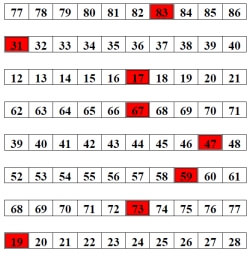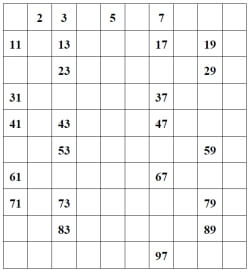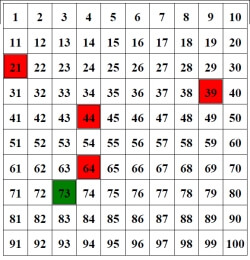Worksheets and No Prep Teaching Resources
Math Worksheets

# Least Common Multiple Worksheets

Factoring in all aspects is an essential math skill, and these in-depth worksheets will give students systematic practice of factorization, as well as comprehension of prime and composite numbers. Greatest common factor and least common factor problems will help students master this skill. This worksheet set also includes open-ended number theory questions, as well as customizable number theory review printables, so students get exactly what they need most.

Prime NumbersFill-in all of the prime numbers up to 100 Color in the prime numbers up to 100 (5 are given) Color in the prime numbers up to 100 (2 are given) Rows - Color in the prime numbers (1 given in each row) Rows - Color in the prime numbers

Composite NumbersFill-in all of the prime numbers up to 100 Color in the prime numbers up to 100 (5 are given) Color in the prime numbers up to 100 (2 are given) Rows - Color in the prime numbers (1 given in each row) Rows - Color in the prime numbers

Prime or Composite NumbersColor in the prime numbers up to 100 (5 are given) Color in the prime numbers up to 100 (2 are given) Rows - Color in the prime numbers (1 given in each row) Rows - Color in the prime numbers

Number Theory
Prime or composite
Divisibility: complete the table
Divisibility: fill in the missing digit
List all of the factors
Prime factorization
Greatest common factor
Least common multiple
Open-ended number theory questions
Number theory review printable

Have a suggestion or would like to leave feedback?